You are currently offline. Some features of the site may not work correctly.

# K-trivial set

Known as: K-trivial sets
In mathematics, a set of natural numbers is called a K-trivial set if its initial segments viewed as binary strings are easy to describe: the prefix… Expand
Wikipedia

## Papers overview

Semantic Scholar uses AI to extract papers important to this topic.
2017
2017
• 2017
• Corpus ID: 11917126
We study the sets that are computable from both halves of some (Martin-Lof) random sequence, which we call \emph{$1/2$-bases}. We… Expand
•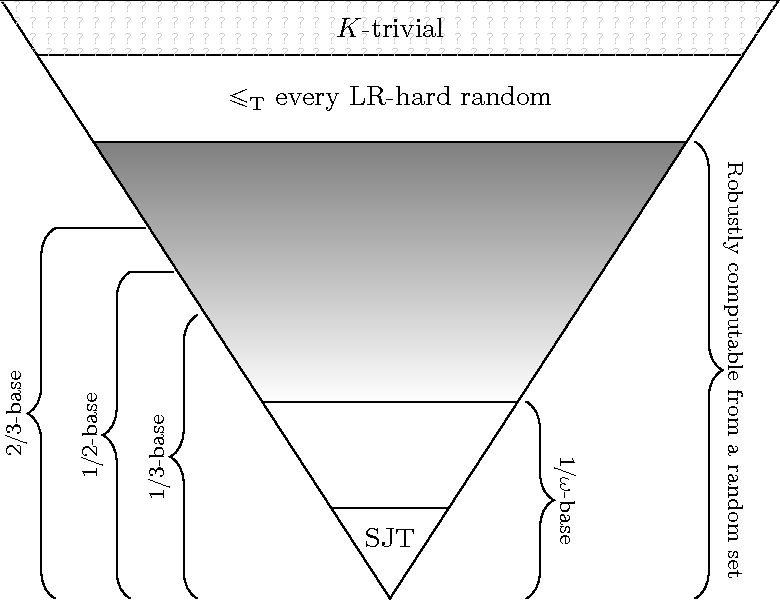•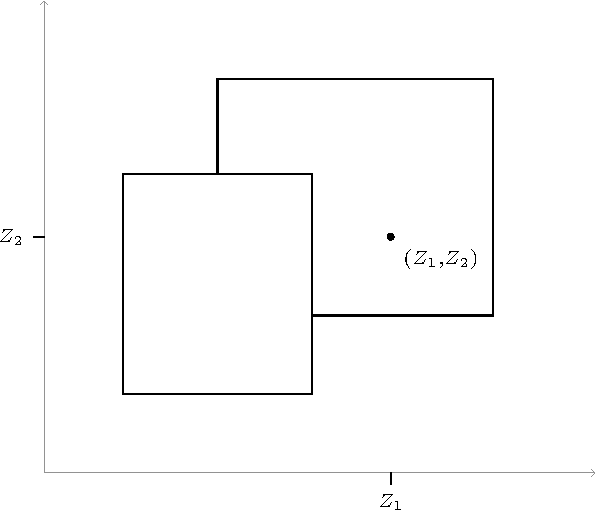Is this relevant?
2016
2016
• 2016
• Corpus ID: 51730106
We show that a Martin-Lof random set for which the effective version of the Lebesgue density theorem fails computes every K… Expand
Is this relevant?
2014
2014
• J. Symb. Log.
• 2014
• Corpus ID: 14951494
The K-trivial sets form an ideal in the Turing degrees, which is generated by its computably enumerable (c.e.) members and has an… Expand
Is this relevant?
2013
2013
I discuss part of the solution for the ML-covering problem . This passes through analytic notions such as martingale… Expand
Is this relevant?
2012
2012
Monotone complexity, Km, is a variant of Kolmogorov complexity that was introduced independently by Levin and Schnorr. The… Expand
Is this relevant?
2011
2011
The field of algorithmic randomness is concerned with making precise the intuitive notion of the randomness of individual objects… Expand
Is this relevant?
2009
2009
• Theor. Comput. Sci.
• 2009
• Corpus ID: 15138081
The sequences which have trivial prefix-free initial segment complexity are known as K-trivial sets, and form a cumulative… Expand
•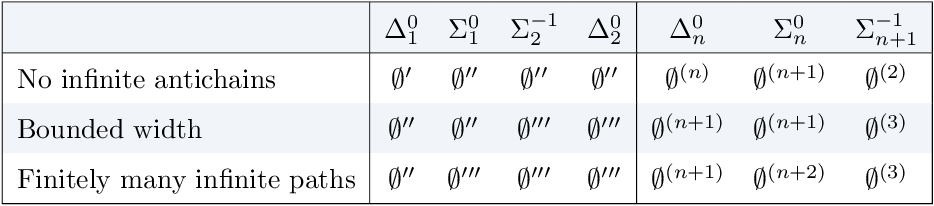•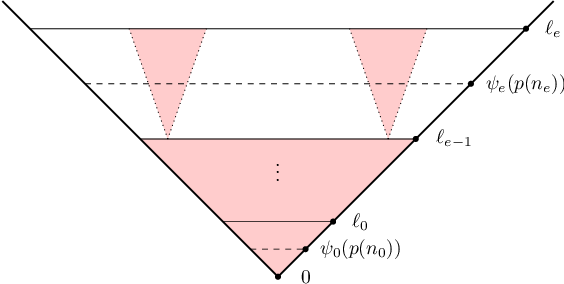•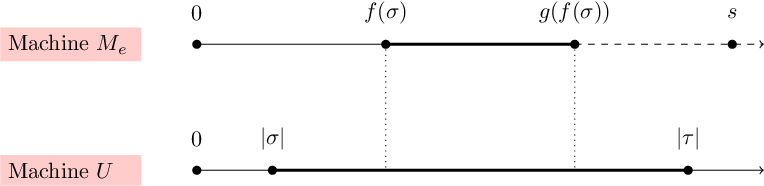Is this relevant?
2008
2008
A real is Martin-Löf (Schnorr) random if it does not belong to any effectively presented null $${\Sigma^0_1}$$ (recursive) class… Expand
Is this relevant?
Highly Cited
2007
Highly Cited
2007
• 2007
• Corpus ID: 9180151
AbstractLet R be a notion of algorithmic randomness for individual subsets of ℕ. A set B is a base for R randomness if there is a… Expand
Is this relevant?
2005
2005
• CiE
• 2005
• Corpus ID: 39007433
For given real α ∈ {0,1}∞, a presentation V of α is a prefix-free and recursively enumerable subset of {0,1}* such that \$\alpha… Expand
Is this relevant?